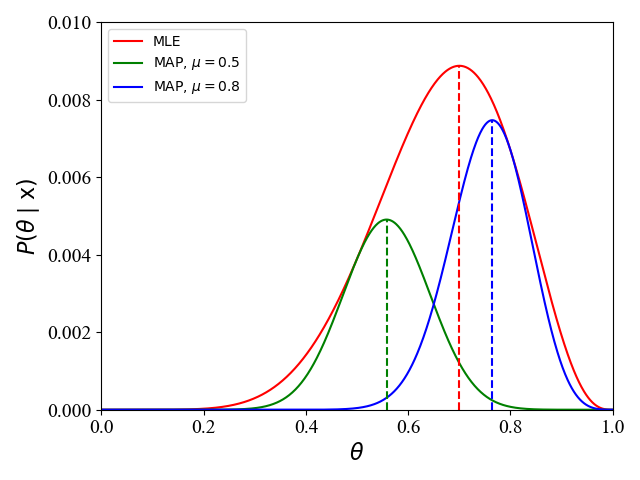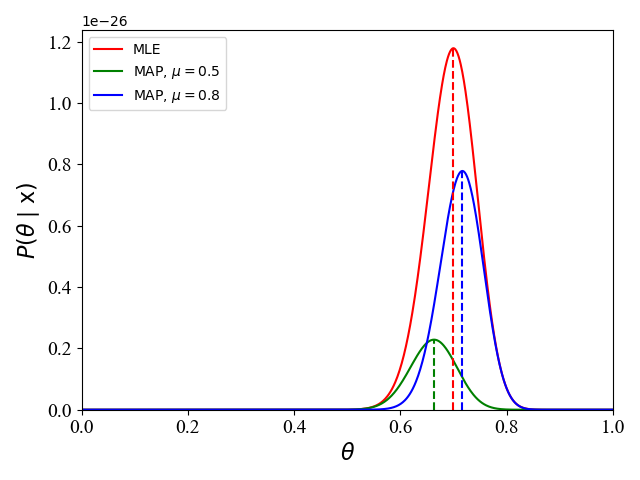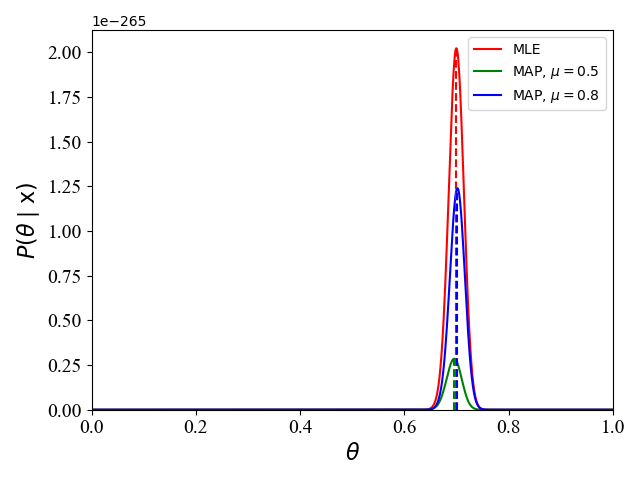0%

• 频率学派 VS. 贝叶斯学派

• 频率学派：事件本身是服从某种参数$\theta$固定的分布。频率学派认为概率即是频率，某次得到的样本$\mathrm x$只是无数次可能的试验结果的一个具体实现，样本中未出现的结果不是不可能出现，只是这次抽样没有出现而已。在参数估计中，频率学派的代表是最大似然估计 MLE。
• 贝叶斯学派：参数$\theta$也是随机分布的。贝叶斯学派认为只能依靠得到的样本$\mathrm x$去做推断，而不能考虑那些有可能出现而未出现的结果。同时，贝叶斯学派引入了主观的概念，认为一个事件在发生之前，人们应该对它是有所认知，即先验概率$p(\theta)$，然后根据样本$\mathrm x$ 通过贝叶斯定理来得到后验概率$p(\theta\mid\mathrm x)$。在参数估计中，贝叶斯学派的代表是最大后验概率估计 MAP。
• 概率 VS. 统计

• The basic problem of probability is: Given the distribution of the data, what are the properties (e.g. its expectation) of the outcomes (i.e. the data)?

• The basic problem of statistical inference is the inverse of probability: Given the outcomes, what can we say about the process that generated the data?

对于在机器学习中的常见问题，这里的data就是我们的训练样例，且机器学习的目的就是 say about the process that generated the data, 即学习生成训练样例的模型。

• 似然函数 VS. 概率函数
似然函数和概率函数的数学表达式一样，只是以不同的角度看待该函数表达式：

• 若$\theta$已知，$\mathrm x$是变量，$P(\mathrm x\mid\theta)$ 被称为概率函数；
• 若$\mathrm x$已知，$\theta$是变量，$P(\mathrm x\mid\theta)$ 被称为似然函数；

​ 一般为了保持符号的一致性，似然函数也经常写作$L(\theta\mid\mathrm x)$。

## 极大似然估计 (MLE)

MLE就是寻找最优的$\theta$最大化公式(2)的概率，即求解

## 最大后验概率估计 (MAP)图1

## 等效情况图2图3